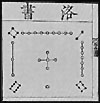# magic matrices: mathematical contexta representation of the oldest known magic square, the Chinese Lo-Shu (3x3)

Magic matrices (including squares, cubes and hypercubes) involve three areas of mathematics:

• Factor analysis
• Matrices
• Combinatorics

of which, the latter two are most relevant to the research behind cubelife.

Factor analysis is concerned with finding and putting things together to give a specified product, i. e. magic squares and cubes.

Matrices are simply defined as a square array of numbers. Magic squares and cubes are such arrays of numbers.

The branch of mathematics devoted to the solution of problems of choosing and arranging the elements of certain (usually finite) sets according to prescribed rules is called combinatorics. The birth of combinatorial analysis as a branch of mathematics is associated with the work of B. Pascal and P. de Fermat on the theory of games of chance. These works, which formed the foundations probability theory, contained principles for determining the number of combinations of elements in a finite set, and established the traditional connection between combinatorial analysis and probability theory. L. Euler contributed greatly to the development of combinatorics. He laid down the beginning of the basic methods of calculating combinatorial configurations.

I began more detailed ongoing work on mapping magic square patterns around 2002, and delivered some findings in presentations around 2021. Older research (on matrix positions) is also at cubelife.org.